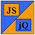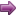# JavaScript & jQuery Tutorials

JavaScript & jQuery Quizzes HomeJavaScript Intermediate Quiz

## JavaScript Intermediate Quiz

The questions in this second quiz on JavaScript 1.5 are on the topics covered in the JavaScript Intermediate section of the site. The table below lists the lessons, a description of the lesson content and the the quiz question number range.

### Lesson Summary

Click on a lesson in the table to go to that lesson for a refresher on the topics for that lesson.

JavaScript 1.5 Intermediate Lessons Description Question Range
Lesson 1 - ArraysIn this lesson we learn about arrays and the array methods used for manipulating our arrays.1 - 4
Lesson 2 - Dates & TimesIn this lesson we learn about dates and times and the methods used for manipulating them.5 - 7
Lesson 3 - Conditional StatementsIn this lesson we take a first look at conditional statements with the `if....else` statement.8 - 10
Lesson 4 - While and Do....While LoopsIn this lesson we take a first look at loops with the while and `do....while` statements.11 - 13
Lesson 5 - For LoopsIn this lesson we take a look at the `for` loop construct and its usage.14 - 16
Lesson 6 - More Maths FunctionsIn this lesson we look at some more maths functions that are available with JavaScript.17 - 20
Lesson 7 - Object LiteralsIn this tutorial we learn about object literals and JSON notation.21 - 23
Lesson 8 - FunctionsIn this lesson we learn about functions and the various ways to create them.24 - 26
Lesson 9 - Regular ExpressionsSummarizing the tutorials in this section.27 - 29

### JavaScript 1.5 Quiz

The quiz below tests your knowledge of the material learnt in the JavaScript Intermediate section of the site.

Question 1 : What happens when we try to create an array without passing any arguments?
- When no arguments are passed an empty array is created.
Next >>
Question 2 : When we create an array with one argument and the argument is not a number what happens?
- When we create an array with one argument and the argument is not a number an array is created with a length of 1, with the argument as the first elements value.
<< Prev
Next >>
Question 3 : On array creation when one argument is passed and it is a number between 0 and 4,294,967,295 an array is created with that length. What happens when a number outside these parameters is passed?
- When one argument is passed and it is a number outside the parameter range 0 and 4,294,967,295 a <code>RangeError</code> exception is thrown.
<< Prev
Next >>
Question 4 : When creating an array using array literal syntax what symbols are used?
- When creating an array using array literal syntax we use the <code>[]</code> symbols.
<< Prev
Next >>
Question 5 : Date creation using the milliseconds parameter starts from which date.?
- Date creation using the milliseconds parameter starts from 1 January 1970 00:00:00 UTC.
<< Prev
Next >>
Question 6 : Date creation using the milliseconds parameter can use negative as well as positive values?
- Date creation using the milliseconds parameter can use negative as well as positive values.
<< Prev
Next >>
Question 7 : Is the following code a valid way of creating a Date object?
`var aDate = new Date(Jan 1, 2000);`
- Yes we can parse a date when creating a Date instance.
<< Prev
Next >>
Question 8 : We must always use an `else` with an `if` statement?
- We can have an <code>if</code> without an <code>else</code>.
<< Prev
Next >>
Question 9 : What is output from the following conditional statement?
```var aVariable = false; if (aVariable = true) { alert('true'); } else { alert('false'); }```
- We are actually assigning <code>true</code> in the <code>if</code> statement. To compare values we need to use <code>==</code>
<< Prev
Next >>
Question 10 : We can have an `if` statement with mutiple `if....else` statements within it?
- An <code>if</code> statement can contain mutiple `if....else` statements within it.
<< Prev
Next >>
Question 11 : Which of these loop statements will always execute at least once?
- The <code>do....while</code> statement will always execute at least once.
<< Prev
Next >>
Question 12 : Which statement allows us to exit a loop?
- The <code>break</code> statement allows us to exit a loop.
<< Prev
Next >>
Question 13 : Which statement allows us to carry on from the expression part of a loop?
- The <code>continue</code> statement allows us to continue from the expression part of the loop.
<< Prev
Next >>
Question 14 : What would happen if we tried to run the following `for` loop code?
```for (;;) { alert('In loop'); }```
- The <code>for</code> loop code loops endlessly.
<< Prev
Next >>
Question 15 : How many times will the following `for` loop execute?
```for (var i=0; i<3; i++) { alert('The i variable is = ' + i); }```
- The <code>for</code> loop code executes 3 times.
<< Prev
Next >>
Question 16 : We can use `break` statements within our `for` loops?
- Yes we can use The <code>break</code> statements within our `for` loops.
<< Prev
Next >>
Question 17 : What is generally returned from functions when a numerical value can't be derived or is unobtainable?
- <code>NaN</code> is generally returned from functions when a numerical value can't be derived or is unobtainable.
<< Prev
Next >>
Question 18 : What is alerted from the following code?
```nanResult = NaN == NaN; alert(nanResult);```
- The Nan global property is also unique in JavaScript in the fact that you cannot rely on the equality (==) and strict equality (===) comparison operators to find out whether a value is Nan or not.
<< Prev
Next >>
Question 19 : What is the `parseFloat()` function used for?
- The <code>parseFloat()</code> function is used to convert a string to a decimal.
<< Prev
Next >>
Question 20 : Care should be taken when using the `IsNaN()` function as results can be unexpected.?
- Parsing a string that cannot be converted to a numeric gives a double positive and makes <code>isNaN()</code> unreliable in this situation.
<< Prev
Next >>
Question 21 : What does the acornym `JSON` stand for?
- <code>JSON</code> is an acornym for JavaScript Object Notation.
<< Prev
Next >>
Question 22 : When using the `JSON` style of coding what symbols do we use to mark object boundaries?
- The <code>JSON</code> style of coding uses matching braces to mark object boundaries.
<< Prev
Next >>
Question 23 : One advantage of using JSON is that code is more compact, what's another advantage?
- The advantages of using JSON is that code is more compact and visually more informative of object structure.
<< Prev
Next >>
Question 24 : Which is the least efficient way to create a function?
- Using the <code>Function</code> constructor to create a function is the least efficient way to create functions as they are parsed on function creation as opposed to being parsed with the rest of The JavaScript code..
<< Prev
Next >>
Question 25 : What method can we use for function chaining?
- We can use both the <code>apply()</code> and <code>call()</code> methods for function chaining.
<< Prev
Next >>
Question 26 : What statement is used to pass a value back from a function?
- The <code>return</code> statement is used to pass a value back from a function.
<< Prev
Next >>
Question 27 : What symbol do we use to escape special characters when using the `RegExp` constructor?
- We use the <code>\</code> (backslash symbol) to escape special characters when using the <code>RegExp</code> constructor.
<< Prev
Next >>
Question 28 : We have a choice of 3 flags to use with regualr expressions and all are mutually exclusive?
- Flags can be used individually or in combination and are applied to the characters we are searching with the regular expression pattern. .
<< Prev
Next >>
Question 29 : Is the following code a valid way to create a regular expression?
`var newRegExp = /\d/;`
- The code is an example of creating a regular expression using lieral format and is perfectly valid.
<< Prev
Finish!
Quiz Progress Bar Please select an answer

### What's Next?

In the next quiz we test our knowledge of the topics covered in the JavaScript Advanced section of the site .